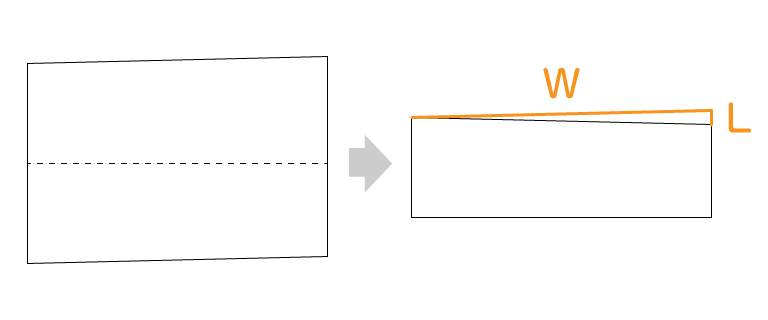SmartDIYs Creator Manual

# Calculation method of correction value

This section describes the "correction" item of the user setting. This correction item is an adjustment value effective to improve the following phenomena.

• Workpiece dimensions different from data
• X axis and Y axis are not perpendicular

## Workpiece dimensions different from data

### Major cause

1. Optical axis adjustment is not appropriate
2. Adjustments such as pulleys and timing belts are not appropriate

If improvement cannot be achieved even if the above adjustment is made, improvement can be expected by setting the size correction item.

### Calculation method of correction value

1. Prepare rectangular processed data of any width and height.
2. We process engraving on materials that resist wrinkles and distortion.
3. The ratio is calculated from the size of data and the size of engraving process.

#### [Example of calculation]

Size of data: H: 200.00 mm x W: 200.00 mm
Actual processing size: H: 199.10 mm x W: 199. 50 mm

[H correction value]

``````How to obtain: (Data) 200.00 / (Actual) 199.10 = 1.00452034 ...
Setting value: 100.45203 (%)
``````

[W correction value]

``````How to obtain: (Data) 200.00 / (Actual) 199.50 = 1.00250626...
Setting value: 100.25063(%)
``````

## Right angle data is not accurate

### Major cause

Cause:

1. Optical axis adjustment is not appropriate
2. There is a tilt when assembling the frame or X axis

If improvement cannot be achieved even if the above adjustment is made, improvement can be expected by adjusting the item of tilt correction.

### Calculation method of correction value

First, if the above "size correction" is completed, the correction value can be calculated more accurately.

1. Prepare rectangular processing data of any width and height.
2. We cut and process materials such as paper without wrinkles.
3. Fold them so that the lower left corner and the upper left corner overlap.
4. If there is a gap between the lower right corner and the upper right corner, measure the distance (L) of this gap.
5. Apply the following formula to calculate the angle.

`θ (deg) = arcsin ( (L/2) / W ) * 180 / π`#### [Example of calculation]

Size of data: H:280.00mm x W:400.00mm
Gap between lower right corner and upper right corner when folded: L: 1.50 mm

[Angle correction value]

``````
Method: arcsin (0.75 / 400.00) * 180 / π
Setting value:
To correct diagonally right up:-0.10743 °
To correct diagonally right down: + 0.10743 °``````

#### ※Please use this automatic calculation function

W length: mm
L length: mm

The correction value is ±

Top